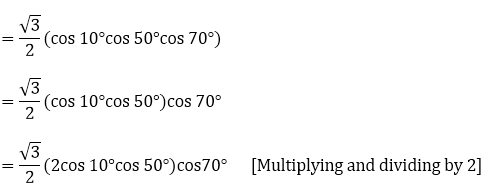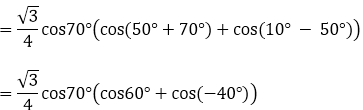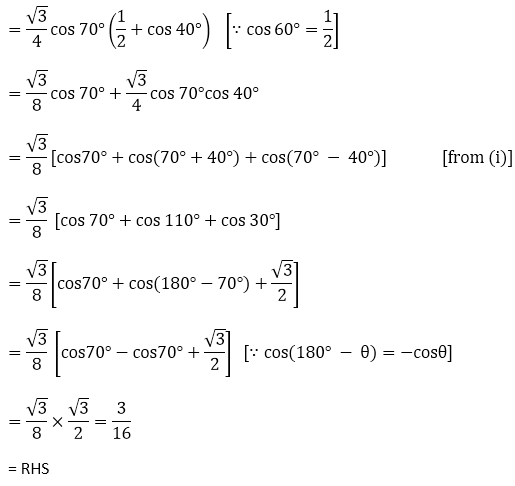#### Thank you for registering.

One of our academic counsellors will contact you within 1 working day.

Please check your email for login details.

Click to Chat

1800-1023-196

+91 7353221155

CART 0

• 0
MY CART (5)

Use Coupon: CART20 and get 20% off on all online Study Material

ITEM
DETAILS
MRP
DISCOUNT
FINAL PRICE
Total Price: Rs.

There are no items in this cart.
Continue Shopping

# Chapter 8: Transformation Formulae – Exercise 8.1

## Transformation Formulae – Exercise 8.1 – Q.1

(i) 2 sin3θ cosθ

= sin(3θ + θ) + sin(3θ - θ) [∵ 2sinA cosB = sin(A + B) + sin(A - B)]

= sin4θ + sin2θ

(ii) 2 cos3θ sin2θ

∵ 2 cosA sinB = sin(A + B) - sin(A - B)

⟹ 2 cos3θ sin2θ = sin(3θ + 2θ) - sin(3θ - 2θ)

= sin5θ - sinθ

(iii) 2 sin4θ sin3θ

∵ 2 sin4θ sin3θ = cos(4θ - 3θ) - cos(4θ + 3θ)

= cosθ - cos7θ

(iv) 2 cos7θ cos3θ

∵ 2 cosA cosB = cos(A + B) + cos(A - B)

⟹ 2 cos7θ cos3θ = cos(7θ + 3θ) + cos(7θ - 3θ)

= cos10θ  + cos4θ

## Transformation Formulae – Exercise 8.1 – Q.2## Transformation Formulae – Exercise 8.1 – Q.3(i)Now,## Transformation Formulae – Exercise 8.1 – Q.3(ii)

LHS = sin 25° cos 115°We know that

2 sin A cos B = sin (A + B) + sin (A - B)Also,

cos θ = sin(90° - θ)

cos 50° = sin(90°- 50°) = sin 40°

=1/2 [sin 40°- 1]

## Transformation Formulae – Exercise 8.1 – Q.4

We have,= -cos θ + [cos (θ + 2θ) + cos(2θ - θ)]

= -cos θ + cos 3θ + cos θ

= cos 3θ

= RHS

∴  LHS = RHS Hence Proved.

## Transformation Formulae – Exercise 8.1 – Q.5(i)

cos 10° cos 30° cos 50° cos 70° = 3/16

LHS = cos10° cos 30° cos 50° cos70°

= cos 30° cos 10° cos 50°cos 70°Also,

⟹ 2cosA cos B = cos(A + B)+ cos(A - B)           --- (i)Now,## Transformation Formulae – Exercise 8.1 – Q.5(ii)

cos 40°cos 80°cos 160° = -1/8

LHS = cos 40°cos 80°cos 160°

= cos 80°cos 40°cos 160°

Multiplying and dividing by 2

=1/2 (cos 80°×(2cos 40°cos 160°))

2cosAcosB = cos(A + B) + cos(A - B)

=1/2 (cos 80°(cos(40°+160°)+cos(40° - 160°)))

=1/2 (cos 80°(cos(40° + 160°)+ cos(-120)))

=1/2 cos 80°(cos(180° + 20°)+ cos(180° - 60°))

=1/2 cos 80°(cos 20°+cos 60°)

=1/2 cos 80°cos 20°+1/2  cos ⁡80°+cos 60°

= – 1/2 (2cos 80°cos 20°)+1/2 cos 80°cos 60°

= – 1/4[2 cos 80°cos 20° + cos 80°]

= – 1/4[cos(80° + 20°) + cos(80° - 20°)+ cos 80°]

= – 1/4[cos100° + cos 60° + cos 80°]

= – 1/4[cos(180° - 80°) + cos 60° + cos 80°]

= – 1/4[-cos 80° + cos 60° + cos 80°]

= – 1/4cos 60°

= – 1/4 × 1/2

= – 1/8 RHS

## Transformation Formulae – Exercise 8.1 – Q.5(iii)

sin 20°sin 40°sin 80°### Course Features

• Video Lectures
• Revision Notes
• Previous Year Papers
• Mind Map
• Study Planner
• NCERT Solutions
• Discussion Forum
• Test paper with Video Solution